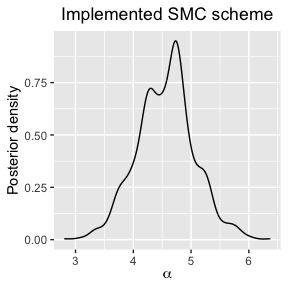# SMC-Mallows Tutorial

#### 2021-10-25

This vignette describes the SMC-Mallows functions of the package. These use Sequential Monte Carlo (SMC) algorithms to provide updated approximations to the posterior distribution of a single Mallows model. We consider scenarios where we receive sequential information in the form of complete rankings, partial rankings and updated rankings from existing individuals who have previously provided a (partial) ranking. We use an alternative data augmentation method, called the pseudolikelihood approach, when we are using the footrule and Spearman distance functions instead of using an independent sampler. SMC-Mallow uses functions similar to their base MCMC counterparts in the BayesMallows package, to visualise and analyse the posterior distributions.

### SMC-Mallows functions

Function Name Description
smc_mallows_new_users Runs the SMC algorithm for case where we observe full rankings as new observational data.
smc_mallows_new_item_rank Runs the SMC algorithm for case where we observe updated partial rankings as from existing users.
plot Plots posterior density of $$\boldsymbol{\rho}$$ or $$\alpha$$ for a selection of items.
compute_posterior_intervals_rho Computes the Bayesian posterior intervals for $$\boldsymbol{\rho}$$.
compute_consensus_rho Computes the CP estimate or MAP estimate of the latent ranks.
compute_posterior_intervals_alpha Computes the Bayesian posterior intervals for $$\alpha$$.

## Introduction

We provide a summary on the Bayesian Mallows model and the proposed Sequential Monte Carlo framework which updates the parameter estimates of the posterior each time we receive new observations for a fixed computational cost. More information on the Bayesian Mallows model can found in Vitelli et al. (2018) and Liu et al. (2019), and a vignette on the BayesMallows R package can be found in Sørensen et al. (2020). A general discussion on SMC can be found in Del Moral, Doucet, and Jasra (2006) and Doucet and Johansen (2009).

### Notation

We have set of $$m$$ distinct items, labelled $$\mathcal{A} = \{A_1, \dots, A_m \}$$, and we are asked to rank these items in order of personal preference with respect to some attribute. This information can be expressed as a ranking $$\boldsymbol{R} = \{ R_1 , \dots , R_m\}$$, which is a mapping $$\boldsymbol{R}:\mathcal{A} \rightarrow \mathcal{P}_m$$, where $$\mathcal{P}_m$$ is the space of $$m$$-dimensional permutations of $$\{1, \dots, m\}$$. Each $$R_i \in \{1,\dots,m\}$$ corresponds to the rank of an item $$A_i$$. We fundamentally assume that the preference information we receive from a group of individuals is transitive, i.e., each individual does not contradict themselves when specifying their preferences. In other words, for any three distinct items $$\{A_i,A_j,A_k\}$$ in a set, then if $$A_i \prec A_j$$ and $$A_j \prec A_k$$, then it must follow that $$A_i \prec A_k$$. Sometimes, we are unable to provide full rankings, so instead we provide a ranking for a subset of the items in $$\mathcal{A}$$. These are referred to as partial rankings. Partial rankings can occur either randomly or an individual can specify their top-$$k$$ ranked items. In this scenario, we will need perform data augmentation in order to estimate the parameters of the Mallows model.

### The Bayesian Mallows Model

The Mallows model is a probability distribution for ranking data. The probability of observing a ranking $$\boldsymbol{R}$$ is defined as

$p(\boldsymbol{R}) = p(\boldsymbol{R}|\boldsymbol{\rho},\alpha) =\frac{1}{Z_m(\alpha)} \exp \lefts\{ -\frac{\alpha}{m} { d(\boldsymbol{R}, \boldsymbol{\rho})} \right\},$

where: $$\boldsymbol{\rho} \in \mathcal{P}_m$$ is the consensus ranking; $$\alpha > 0$$ is the scale parameter which represents the variability in rankings within the group of individuals around the consensus ranking; and $$Z_m(\alpha)$$ is the normalisation constant. The distance function, $$d(\cdot,\cdot) : \mathcal{P}_m \times \mathcal{P}_m \rightarrow [0,\infty)$$, measures the ‘’closeness’’ of a ranking to the consensus ranking. The Mallows literature discusses the use of a right-invariant distance function, which means that the distance between two items is unaffected by relabelling of items . The distance metrics that the BayesMallows R package currently uses are: footrule, Spearman, Cayley, Kendall and Hamming. This also means that the normalisation constant is independent of the consensus ranking.

Vitelli et al. (2018) extended the Mallows model to incorporate a Bayesian framework for inference. A uniform prior is elicited for the consensus ranking $$\pi(\boldsymbol{\rho}) = (m!)^{-1} 1_{\mathcal{P}_m} (\boldsymbol{\rho})$$ in the space of $$\mathcal{P}_m$$, and an exponential prior for $$\alpha$$, with density $$\pi(\alpha|\lambda) = \lambda \exp \{ -\lambda \alpha \} 1_{[0,\infty)}(\alpha)$$. Given $$M$$ observed complete rankings and the prior distributions $$\pi(\boldsymbol{\rho})$$ and $$\pi(\alpha)$$, assuming prior independence of these variables, we have the following posterior density, known as the Bayesian Mallows model,

$\pi(\boldsymbol\rho, \alpha | \boldsymbol{R}_1, \dots, \boldsymbol{R}_M) \propto \frac{\pi(\boldsymbol\rho)\pi(\alpha) }{[Z(\alpha)]^M} \exp \left\{ - \frac{\alpha}{m} \sum_{j=1}^{M} d(\boldsymbol{R}_j, \boldsymbol\rho ) \right\}.$

Any posterior estimates of interest, such as the marginal posterior for $$\boldsymbol{\rho}$$, are obtained through the use of Metropolis-Hastings based Markov Chain Monte Carlo (MCMC) algorithm. Full details of the algorithm can be found in Vitelli et al. (2018). In each iteration of the algorithm, a new consensus ranking $$\boldsymbol{\rho}'$$ is proposed to update $$\boldsymbol{\rho}$$ according to a distribution which is centered around the current rank $$\boldsymbol{\rho}$$. The proposal step for $$\boldsymbol{\rho}$$ is done using the leap-and-shift proposal algorithm of Vitelli et al. (2018) and a new value $$\alpha'$$ is sampled from the log-normal distribution to update the current value of $$\alpha$$.

Inference on the Bayesian Mallows model can sample the posterior distribution of the unknown consensus ranking and scale parameter using a variety of observed data including: full rankings, incomplete rankings (e.g. top-$$k$$ rankings and ranks missing at random), and implicit data such as pairwise comparisons. For example, in the case of partial rankings, we can create augmented full ranking $$\tilde{R}_1, \dots, \tilde{R}_M$$ by using an independent sampler for each assessor containing the set of rankings not already chosen. The MCMC algorithm alternates between sampling a new value of $$\boldsymbol{\rho}$$ and $$\alpha$$ given the current $$\tilde{R}_1, \dots, \tilde{R}_M$$ and sampling $$\tilde{R}_1, \dots, \tilde{R}_M$$ given the current values of $$\boldsymbol{\rho}$$ and $$\alpha$$. The existing methods are discussed in Vitelli et al. (2018) and are provided in the BayesMallows R package .

### Sequential Monte Carlo

Sequential Monte Carlo (SMC) methods are a class of sampling algorithms which are used to estimate a sequence of target distributions given a stream of observations over discrete time. Each target distribution is approximated by a collection of random samples, termed particles, at each time step and evolve according to importance sampling and resampling steps. The literature on SMC methods is vast and diverse, but we are interested in using SMC as an alternative to MCMC methods . A nice summary of the different variants of SMC is given in Del Moral, Doucet, and Jasra (2006).

In SMC, the aim is to approximate a sequence of target distributions $$\pi_t(\boldsymbol{\theta})$$ with parameters $$\boldsymbol{\theta}$$ that we wish to estimate given a set of observed data $$D_t$$ which has accumulated up to time $$t$$. So we can write a target distribution $$\pi_t$$ as a posterior distribution

$\pi_t(\boldsymbol{\theta}) = \pi_t(\boldsymbol{\theta} | D_t) \propto \pi_0(\boldsymbol{\theta})p_t(D_t| \boldsymbol{\theta}).$

The SMC algorithm begins by generating $$N$$ particles using the prior distributions for each parameter and assigning each particle an equal weight. In each time step $$t=1,\dots,T$$, we assume that an additional $$p$$ independent observations $$y_{1:p}$$ become available from the target distribution. We reweight the particles in $$\pi(\boldsymbol{\theta}| D_{t-1})$$ from time $$t-1$$ to $$t$$ such that they are weighted with respect to $$\pi(\boldsymbol{\theta}| D_{t})$$,

$w^{(i)}_t = \frac{\pi(\boldsymbol{\theta}^{(i)}_{t-1} | D_{t})}{\pi(\boldsymbol{\theta}^{(i)}_{t-1} | D_{t-1})} \propto \frac{p(D_t | \boldsymbol{\theta}^{(i)}_{t-1})}{p(D_{t-1} | \boldsymbol{\theta}^{(i)}_{t-1})} = p( y_{1:p}| D_{t-1}, \boldsymbol{\theta}_{t-1}^{(i)} ), \ i = 1,\dots,N.$

Next, we normalise the particle weights and resample the particles with replacement which replicates the heavier weighted particles and discard those with negligible weights. This results in a set of equally weighted particles $$\{ \boldsymbol{\theta}_t^{(i)}, w_t^{(i)} = \frac{1}{N} \}_{i=1}^N$$ that represent a sample of the posterior distribution. A summary of the possible methods for resampling is given in Doucet and Johansen (2009).

In the final stage, we move the particles using an MCMC kernel within SMC after resampling to give back the diversity of particle values . This particular methodology in SMC is often referred to as the Resample-Move framework of Berzuini and Gilks (2001) and Berzuini and Gilks (2003). We can apply MCMC kernel many times as we would like since the particles are still moving within the stationary distribution $$\pi_t$$.

## SMC-Mallows User Guide

The SMC-Mallows functions contain algorithms to perform the Resample-Move SMC framework of Berzuini and Gilks (2001) using a single Mallows model. Each algorithm begins by drawing $$N$$ particles using the priors for $$\boldsymbol{\rho}$$ and $$\alpha$$ or by using specified initial values. Each particle is also assigned equal weight so at the start of the SMC algorithm we have $$\{\boldsymbol{\theta}^{(i)}_0 = (\boldsymbol{\rho}_0^{(i)}, \alpha_0^{(i)}), w^{(i)} \}_{i=1}^{N}$$ as the set of particles. Next, we observe some ranking data, $$D_t$$, and we calculate the updated weights of the particles with respect to the new observations and their contribution to the current estimated posterior distribution before reweighting and multinomial resampling. Finally, we perturb the particles using the Metropolis-Hastings based MCMC kernel and we use the proposal distributions described in Vitelli et al. (2018) for sampling values of $$\boldsymbol{\rho}$$ and $$\alpha$$.

### Complete Rankings

For this case, we assume that we observe a collection of complete rankings from new assessors over a sequence of discrete time steps, $$t=1,\dots, T$$, such that up to a time $$t$$, we will have observed $$|M_t|$$ complete rankings. The particles are reweighted such that they are representative of the underlying distribution of the $$|M_t|$$ complete rankings. The new weights for each particle are calculated as

\begin{align*} {w}^{(i)}_t(\boldsymbol{\theta}^{(i)}_{t-1}, \boldsymbol{\theta}^{(i)}_{t}) &= \frac{ (Z_m(\alpha^{(i)}_{t-1}))^{-|M_t|} \exp \left\{ - \frac{\alpha^{(i)}_{t-1}}{m} \sum_{j=1}^{|M_t|} d(\mathbf{R}^{(i)}_j, \boldsymbol{\rho}^{(i)}_{t-1} ) \right\} }{ (Z_m(\alpha^{(i)}_{t-1}))^{-|M_{t-1}|} \exp \left\{ - \frac{\alpha^{(i)}_{t-1}}{m} \sum_{j=1}^{|M_t|} d(\mathbf{R}^{(i)}_j, \boldsymbol{\rho}^{(i)}_{t-1} ) \right\} } \\ &= (Z_m(\alpha^{(i)}_{t-1}))^{-(|M_t|-|M_{t-1}|)}\exp \left\{ - \frac{\alpha^{(i)}_{t-1}}{m} \sum_{j= |M_{t-1}|+1}^{|M_t|} d(\mathbf{R}^{(i)}_j, \boldsymbol{\rho}^{(i)}_{t-1} ) \right\} , \end{align*}

where $$\alpha^{(i)}_{t-1}$$ and $$\boldsymbol{\rho}^{(i)}_{t-1}, \ i=1,\dots,N$$ are the current estimated parameter values of the Mallows posterior before we reweight.

#### Demonstration

We are interested in updating the parameter estimates of the Bayesian Mallows model based on the existing ranking data and new observations. We demonstrate the SMC-Mallows functions using the sushi_rankings dataset , which contains 5000 rankings for 10 sushi dishes.

head(sushi_rankings)
##      shrimp sea eel tuna squid sea urchin salmon roe egg fatty tuna tuna roll
## [1,]      2       8   10     3          4          1   5          9         7
## [2,]      1       8    6     4         10          9   3          5         7
## [3,]      2       8    3     4          6          7  10          1         5
## [4,]      4       7    5     6          1          2   8          3         9
## [5,]      4      10    7     5          9          3   2          8         1
## [6,]      4       6    2    10          7          5   1          9         8
##      cucumber roll
## [1,]             6
## [2,]             2
## [3,]             9
## [4,]            10
## [5,]             6
## [6,]             3

The function smc_mallows_new_users with type = "complete" shows the simplest version of the SMC algorithm in action. Many of the input variables, that the function requires, are also used in some of the existing functions in the BayesMallows R package. However, we will discuss what the new variables refer to in the algorithm. The variable R_obs in this instance is a 2D dataset with the number of columns as n_items and the number of rows, $$M$$, represents the number of individuals in the dataset. We begin the algorithm with no observations and in each artificial time step we introduce a batch number of observations, this is controlled by the variable num_new_obs to control the size of the bath, and Time to specify the number of updates we will perform. There is a limit on the value for Time, and this depends on the number of individuals in the dataset and the value of num_new_obs. In the move stage we can apply an MCMC kernel as many times as we would like using the variable mcmc_kernel_app to specify the value.

n_items <- dim(sushi_rankings)
metric <- "footrule"
alpha_vector <- seq(from = 0, to = 15, by = 0.1)
iter <- 1e4
degree <- 10
# Estimate the logarithm of the partition function of the Mallows rank model
logz_estimate <- estimate_partition_function(
method = "importance_sampling",
alpha_vector = alpha_vector,
n_items = n_items, metric = metric,
nmc = iter, degree = degree
)
data <- sushi_rankings[1:100, ]
leap_size <- floor(n_items / 5)
N <- 1000
Time <- 20
smc_test <- smc_mallows_new_users(
R_obs = data, type = "complete", n_items = n_items,
metric = metric, leap_size = leap_size,
N = N, Time = Time,
logz_estimate = logz_estimate,
mcmc_kernel_app = 5,
num_new_obs = 5,
alpha_prop_sd = 0.5,
lambda = 0.15,
alpha_max = 1e6
)

The example smc_test returns a list variable of the particles values for $$\boldsymbol{\rho}$$ and $$\alpha$$ at each time step, so we can observe the posterior as it evolves. Specifically the list contains a three dimensional matrix of size N by n_items by Time+1, named rho_samples, and an N by Time+1 matrix called alpha_samples. These matrices can be studied using some post-processing functions for visualising and analysing the posterior. It is also possible to make comparative results using the MCMC algorithm and the SMC algorithm. Unlike MCMC we cannot observe the trace plots and we do not need to specify a non-zero value for the burn-in. The indexing in R means that we have to view the Time+1 slice of the output in order to view the posterior once all 100 rankings have been observed.

Here, we can observe the posterior probabilities of $$\boldsymbol{\rho}$$ for a selection of items can be observed by calling the function plot().

plot(smc_test, colnames = colnames(sushi_rankings), parameter = "rho")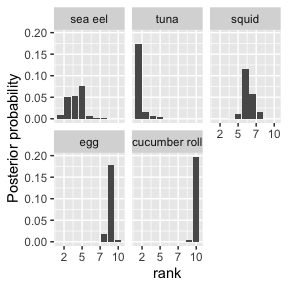The posterior distributions of $$\boldsymbol{\rho}$$ and $$\alpha$$ can be studied with some of the the analysis tools provided by SMC-Mallows. The posterior intervals for the consensus ranking of each sushi item are obtained by calling compute_posterior_intervals_rho.

test_sample_rho <- smc_test$rho_samples[, , Time + 1] compute_posterior_intervals_rho( output = test_sample_rho, nmc = N, burnin = 0, verbose = FALSE ) ## item parameter mean median conf_level hpdi ## Cluster 1.Item 1 Item 1 rho 4 4 95 % [2,5] ## Cluster 1.Item 2 Item 2 rho 4 4 95 % [2,5] ## Cluster 1.Item 3 Item 3 rho 2 2 95 % [2,4] ## Cluster 1.Item 4 Item 4 rho 6 6 95 % [5,8] ## Cluster 1.Item 5 Item 5 rho 8 8 95 % [6,9] ## Cluster 1.Item 6 Item 6 rho 4 4 95 % [3,5] ## Cluster 1.Item 7 Item 7 rho 9 9 95 % [8,9] ## Cluster 1.Item 8 Item 8 rho 1 1 95 %  ## Cluster 1.Item 9 Item 9 rho 7 7 95 % [6,8] ## Cluster 1.Item 10 Item 10 rho 10 10 95 %  ## central_interval ## Cluster 1.Item 1 [2,5] ## Cluster 1.Item 2 [2,6] ## Cluster 1.Item 3 [2,4] ## Cluster 1.Item 4 [5,8] ## Cluster 1.Item 5 [6,9] ## Cluster 1.Item 6 [3,5] ## Cluster 1.Item 7 [8,9] ## Cluster 1.Item 8  ## Cluster 1.Item 9 [6,8] ## Cluster 1.Item 10  We can also rank the sushi items according to their cumulative probability (CP) consensus and their maximum posterior rankings (MAP). These are calculated by calling the function compute_consensus_rho. We demonstrate with the CP consensus: compute_rho_consensus( output = test_sample_rho, nmc = N, burnin = 0, C = 1, type = "CP", verbose = FALSE ) ## ranking item cumprob ## cluster 1 1 Item 8 1.000 ## cluster 11 2 Item 3 0.869 ## cluster 12 3 Item 1 0.460 ## cluster 13 4 Item 2 0.572 ## cluster 14 5 Item 6 0.983 ## cluster 15 6 Item 4 0.627 ## cluster 16 7 Item 9 0.951 ## cluster 17 8 Item 5 0.905 ## cluster 18 9 Item 7 0.984 ## cluster 19 10 Item 10 1.000 Similarly, we can observe the posterior density and the posterior intervals for the scale parameter using the functions plot() and compute_posterior_intervals_alpha. plot(smc_test, nmc = N, burnin = 0, parameter = "alpha")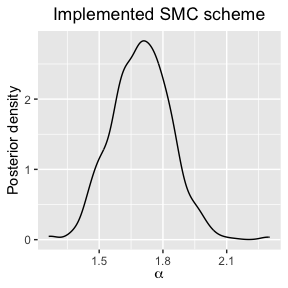test_sample_alpha <- smc_test$alpha_samples[, Time + 1]
compute_posterior_intervals_alpha(
output = test_sample_alpha,
nmc = N, burnin = 0, verbose = FALSE
)
##           parameter mean median conf_level        hpdi central_interval
## Cluster 1     alpha  1.7    1.7       95 % [1.44,1.95]      [1.44,1.97]

### Partial Rankings

#### Pseudolikelihood Sampler

In Vitelli et al. (2018), the augmentation scheme for partially observed rankings is done by using an independent sampler conditioned in the observed component of each ranking. The drawback of this approach is that it does not take into account any existing information, such as the current estimates of the consensus ranking and the scale parameter. We have an option to use an alternative augmentation kernel where, for each item, we use a univariate Mallows distribution to select each rank to an item based on the item’s rank in the estimated consensus ranking and the scale parameter. This approach is similar to the importance sampling approximation of the normalisation constant of the Bayesian Mallows model, full details can be found in Vitelli et al. (2018). This particular augmentation method only applies if we are using the footrule and Spearman distance metric.

#### New Assessors with Partial Rankings

We augment the missing item ranks in each $$\mathbf{R}$$ to create a complete auxiliary ranking $$\tilde{\mathbf{R}}$$ in order to perform the remaining steps of the SMC algorithm. We reweight the particles such that are representative of the underlying distribution of the $$|M_t|$$ augmented rankings. The particle weights are recalculated as

\begin{align*} {w}^{(i)}_t(\boldsymbol{\theta}^{(i)}_{t-1}, \boldsymbol{\theta}^{(i)}_{t}) &= (Z_m(\alpha^{(i)}_{t-1}))^{-(|M_t|-|M_{t-1}|)}\exp \left\{ - \frac{\alpha^{(i)}_{t-1}}{m} \sum_{j= |M_{t-1}|+1}^{|M_t|} d(\tilde{\mathbf{R}}^{(i)}_j, \boldsymbol{\rho}^{(i)}_{t-1} ) \right\} \\ & \times \prod_{j = |M_{t-1}|+1}^{|M_t|} q(\tilde{\mathbf{R}}^{(i)}_j | \mathbf{R}_j, \boldsymbol{\rho}_{t-1}^{(i)}, \alpha_{t-1}^{(i)} ), \end{align*}

where $$\alpha^{(i)}_{t-1}$$ and $$\boldsymbol{\rho}^{(i)}_{t-1}, \ i=1,\dots,N$$ are the current estimated parameter values of the Mallows posterior before we reweight. The distribution $$q()$$ represents the probability of creating an augmented ranking $$\tilde{\mathbf{R}}$$ given the observed ranking $$\mathbf{R}$$ and the current estimated parameters of the posterior.

#### Demonstration

For this demonstration we shall assume that we can only observe the top-5 ranked items from each user in the first 100 rows of the sushi_rankings dataset.

data_partial <- sushi_rankings[1:100, ]
data_partial[data_partial > 5] <- NA
head(data_partial)
##      shrimp sea eel tuna squid sea urchin salmon roe egg fatty tuna tuna roll
## [1,]      2      NA   NA     3          4          1   5         NA        NA
## [2,]      1      NA   NA     4         NA         NA   3          5        NA
## [3,]      2      NA    3     4         NA         NA  NA          1         5
## [4,]      4      NA    5    NA          1          2  NA          3        NA
## [5,]      4      NA   NA     5         NA          3   2         NA         1
## [6,]      4      NA    2    NA         NA          5   1         NA        NA
##      cucumber roll
## [1,]            NA
## [2,]             2
## [3,]            NA
## [4,]            NA
## [5,]            NA
## [6,]             3

We can call the function smc_mallows_new_users with type = "partial" to run the SMC algorithm for partial ranking data. The variable aug_method allows you to choose which data augmentation method to use on the partial ranking data. The option "pseudolikelihood" is only compatible with when selecting the distance metric as either "footrule" or "spearman".

aug_method <- "pseudolikelihood"
metric <- "cayley"
# example of selecting the incorrect combination of metric and aug_method
smc_partial_test <- smc_mallows_new_users(
R_obs = data_partial,
type = "partial",
n_items = n_items,
metric = metric,
leap_size = leap_size, N = N,
Time = Time,
logz_estimate = logz_estimate,
mcmc_kernel_app = 5,
num_new_obs = 5,
alpha_prop_sd = 0.5,
lambda = 0.15,
alpha_max = 1e6,
aug_method = aug_method
)
#>Error in smc_mallows_new_users(R_obs = data_partial, type = "partial",
#>: Combined choice of metric and aug_method is incompatible

This time we call the function again but this time we provide a valid combination of the augmentation method and the distance metric.

aug_method <- "pseudolikelihood"
metric <- "footrule"
smc_partial_test <- smc_mallows_new_users(
R_obs = data_partial,
type = "partial",
n_items = n_items,
metric = metric,
leap_size = leap_size, N = N,
Time = Time,
logz_estimate = logz_estimate,
mcmc_kernel_app = 5,
num_new_obs = 5,
alpha_prop_sd = 0.5,
lambda = 0.15,
alpha_max = 1e6,
aug_method = aug_method
)

The variable smc_test_partial contains a list with three dimensional matrix of size N by n_items by Time+1, named rho_samples, and a N by Time+1 matrix called alpha_samples. The analysis performed in the previous demonstration can be applied to this scenario. Here, we can observe the posterior probabilities for a selection of items in $$\boldsymbol{\rho}$$ and posterior density for $$\alpha$$ as part of this demonstration, but we can use other post processing functions to analyse the output.

plot(smc_partial_test, colnames = colnames(sushi_rankings), parameter = "rho")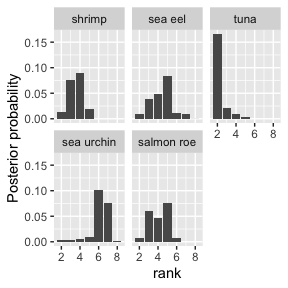plot(smc_partial_test, nmc = N, burnin = 0, parameter = "alpha")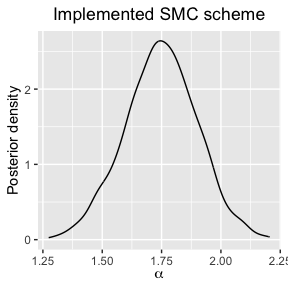### Updated partial rankings

We can view this scenario as a observing an updated partial ranking from an known individual, but a previously latent ranking for an unranked items becomes known. For example, an individual may provided a partial ranking for 6 items $$(1,2,3,\texttt{NA},\texttt{NA},\texttt{NA})$$ and in the SMC algorithm we might have obtained an augmented ranking $$(1,2,3,5,6,4)$$. However, later on the same individual may have provided more information about their ranking, e.g., $$(1,2,3,4,\texttt{NA},\texttt{NA})$$, and this ranking is no longer consistent with the augmented ranking. This causes a problem where we have an augmented complete ranking through SMC, conditioned on the original observed partial ranking, that happens to be no longer consistent with the new observed partial ranking. This means that our algorithm has an additional step before the reweighting stage. In order to reweight the particles correctly as a representative sample of the current posterior, we view the new information arriving into the system as an existing individual (and their augmented ranking) leaving the system completely and then re-entering as a new partial ranking with its extra information. For each individual leaving and re-entering the system, we have to make two weight adjustments to account for these actions.

#### Demonstration

To illustrate how to perform SMC for updated partial rankings, we will modify a much smaller dataset called potato_visual. This dataset, which is described in Liu et al. (2019) and is provided in the BayesMallows R package, represents the rankings given by 12 assessors on 20 potatoes based on how heavy each potato appeared to be given their visual appearance. We create several partial subsets of the complete dataset by removing the lowest ranked item from each assessor from each previous subset, so that we achieve several partial datasets which view the top 10, top 11,… top 19 highest ranked items as well as the complete set of rankings.

example_dataset <- potato_visual
n_users <- 12
n_items <- 20
test_dataset <- array(0, c(n_users, n_items, (n_items / 2 + 1)))
test_dataset[, , (n_items / 2 + 1)] <- potato_visual
tt <- 0
for (ii in (n_items - 1):(n_items / 2)) {
tt <- tt + 1

# set n_users line with one more NA
example_dataset[example_dataset > ii] <- NA

# set as new time stamp
test_dataset[, , ((n_items / 2 + 1) - tt)] <- example_dataset
}

This means that we now have a 3D array containing 10 partial datasets and the one complete dataset. The third dimension of the array represents artificial time. We can view the updated partial rankings scenario as viewing several 2D slices of the observed dataset sequentially. So you can see, for example, that at the 5th time point, we observe the top-14 items from potato_visual.

test_dataset[, , 5]
##       [,1] [,2] [,3] [,4] [,5] [,6] [,7] [,8] [,9] [,10] [,11] [,12] [,13]
##  [1,]   10   NA   NA   NA    6   NA    4   NA    3     5    12     1     2
##  [2,]   10   NA   NA   NA   11   NA    6   NA    4     3    13     1     2
##  [3,]   12   NA   NA   NA   13   11    7   NA    6     3     8     2     1
##  [4,]    9   NA   NA   NA   10   NA    5   NA    3     4     8     1     2
##  [5,]   12   NA   NA   NA    7   NA    2   NA    3     9    13     1     4
##  [6,]   10   NA   NA   NA    8   NA    6   NA    3     7    11     1     2
##  [7,]    9   NA   NA   NA   10   NA    5   NA    3     8    11     1     2
##  [8,]   14   NA   NA   NA   11   NA    6   NA    4     3    10     1     2
##  [9,]    8   NA   NA   NA   12   13    6   NA    5     3     7     1     4
## [10,]    7   NA   NA   NA    9   NA    5   NA    3    10    11     1     2
## [11,]   12   NA   NA   NA   13   NA    7   NA    3     5    11     1     2
## [12,]   14   NA   NA   NA   12   NA    8   NA    3     4     9     1     2
##       [,14] [,15] [,16] [,17] [,18] [,19] [,20]
##  [1,]     9    NA     8     7    14    13    11
##  [2,]     7    NA     8     5    12     9    14
##  [3,]     4    NA     5     9    14    10    NA
##  [4,]     7    NA    11     6    13    14    12
##  [5,]     5    NA    11     6     8    10    14
##  [6,]     4    NA     9     5    13    12    14
##  [7,]     6    NA     7     4    14    12    13
##  [8,]     7    NA     8     5    12     9    13
##  [9,]     2    NA    10     9    NA    14    11
## [10,]     6    NA     8     4    13    12    14
## [11,]     6    NA    10     4    14     8     9
## [12,]     7    NA     6     5    13    10    11

We can now run an experiment with the altered potato_visual dataset by calling the function smc_mallows_new_item_rank.

# Recalculate the estimate of the partition function for 20 items
logz_estimate <- estimate_partition_function(
method = "importance_sampling",
alpha_vector = alpha_vector,
n_items = n_items, metric = metric,
nmc = iter, degree = degree
)
Time <- dim(test_dataset)
N <- 1000
aug_method <- "pseudolikelihood"
metric <- "footrule"
smc_test_updated_partial <- smc_mallows_new_item_rank(
n_items = n_items,
R_obs = test_dataset,
metric = metric,
leap_size = leap_size, N = N,
Time = Time,
logz_estimate = logz_estimate,
mcmc_kernel_app = 5,
alpha_prop_sd = 0.5,
lambda = 0.15,
alpha_max = 1e6,
aug_method = aug_method
)

Unlike the two previous demonstrations, the final slice of the output occurs at Time instead of Time+1. This is because we initialised the algorithm with the first slice of the test_dataset rather than initialising with no observed data. We observe the posterior probabilities for items in $$\boldsymbol{\rho}$$ and posterior density for $$\alpha$$ by using the same post-processing functions as before.

plot(smc_test_updated_partial, parameter = "rho")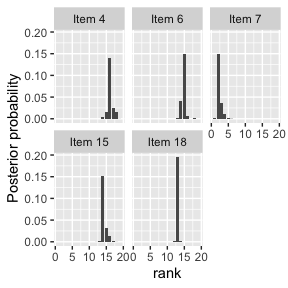plot(smc_test_updated_partial, parameter = "alpha")